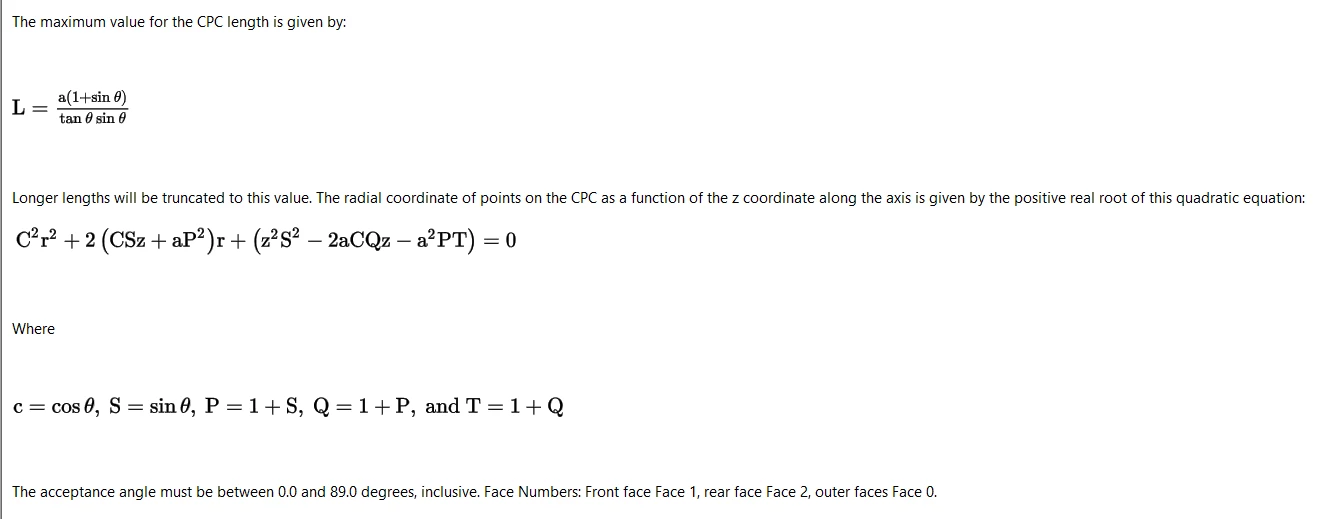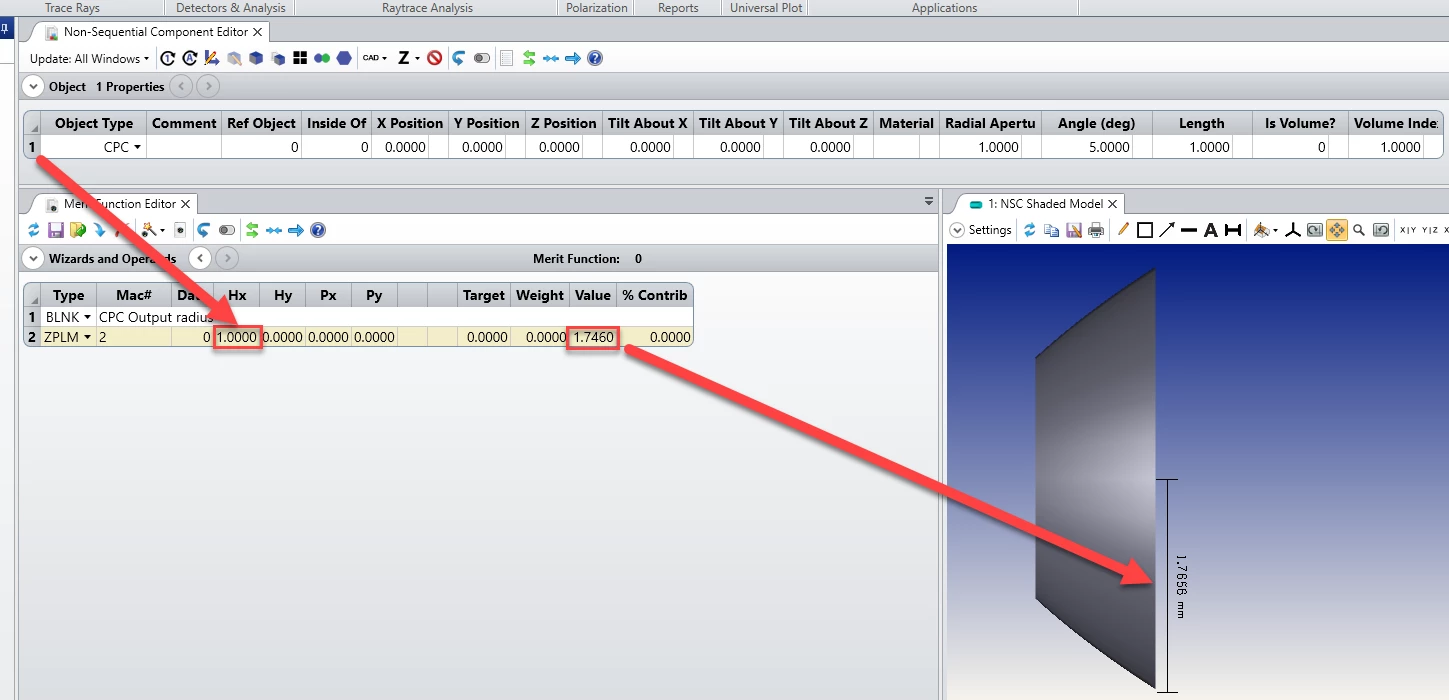Solved

# Output radius of the CPC in the Merit Function

• 3 replies
• 136 views

• Zemax Staff
• 23 replies
I need to constrain the output diameter of the CPC object. However this parameter is not available in the parameters. Do you have a work around for that?
icon

Best answer by David Nguyen 10 October 2019, 17:43

View original

### 3 replies

Yes, we do !

The maths behind the CPC objects are described in the Help File. Retrieving the output diameter implies solving a quadratic equation with the CPC parameters.This sort of tasks is usually addressed through user defined operands that are written with the Zemax Programming Language (ZPL). As described in this knowledge base article.

I’m attaching the ZPL Macro to this thread, and I’ve also copied it here for your reference.

``` # Constants
PI = 3.14159265359
# CPC object number from Hx cell of ZPLM
cpc_number = PVHX()
# === Retrieve CPC parameters === #
aper = NPAR(1, cpc_number, 1)
# Angle (deg)
t = NPAR(1, cpc_number, 2)
t = t/180*PI
# Volume index
is_vol = NPAR(1, cpc_number, 6)
nd     = NPAR(1, cpc_number, 7)
IF (nd > 1)
IF (is_vol > 0)
t = ASIN(SINE(t)/nd)
ENDIF
ENDIF
# Length
L = NPAR(1, cpc_number, 3)
# =============================== #
# === Definitions from CPC Help file === #
C = COSI(t)
S = SINE(t)
P = 1+S
Q = 1+P
T = 1+Q
# ====================================== #
# Maximum length of the CPC
L_max = aper*(1+S)*C/(S*S)
# If current length is longer than maximum length
# change it to the maximum length
IF (L > L_max) THEN (L = L_max)
# Resolution of the quadratic equation for the
A_coeff = C*C
B_coeff = 2*(C*S*L+aper*P*P)
C_coeff = (L*L*S*S-2*aper*C*Q*L-aper*aper*P*T)
delta = SQRT(B_coeff*B_coeff-4*A_coeff*C_coeff)
# Physically meaningful solution

This macro should be placed in the \Documents\Zemax\Macros folder, and can be called in the Merit Function through the ZPLM operand where Mac# is 2, and Hx corresponds to the CPC Object number in the non-sequential component editor.

The result looks as follow for a default CPC:This operand can then be constrained with the OPGT and OPLT operands.